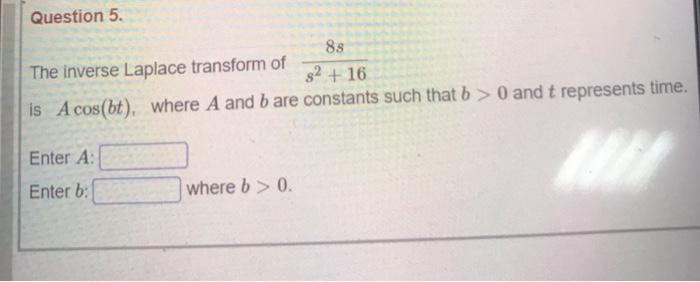### Create an Account

Already have account?

### Forgot Your Password ?

Home / Questions / Question 5. 88 The inverse Laplace transform of S2 + 16 is A cos(bt) where A and b are con...

# Question 5. 88 The inverse Laplace transform of S2 + 16 is A cos(bt) where A and b are constants such that b > 0 and t represents time. Enter A: Enter b: where b > 0.

Question 5. 88 The inverse Laplace transform of S2 + 16 is A cos(bt) where A and b are constants such that b > 0 and t represents time. Enter A: Enter b: where b > 0.Apr 15 2021 View more View Less

#### Answer (Solved)Subscribe To Get Solution